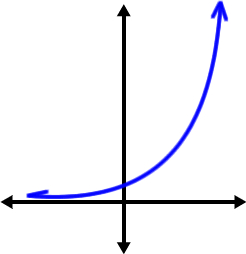# Jointly and cube power

If y varies jointly as x and the cube of z and y=16 when x=4 and z=2, find an equation that represent this relationship

Result

y = (Correct answer is: x z^3)### Step-by-step explanation:Did you find an error or inaccuracy? Feel free to write us. Thank you!